# Second Law Of Thermodynamics

The second law of thermodynamics put restrictions upon the direction of heat transfer and achievable efficiencies of heat engines. The first law of thermodynamics states that the energy of the universe remains constant, though energy can be exchanged between system and surroundings, it can’t be created or destroyed.

While the first law of thermodynamics gives information about the quantity of energy transfer is a process, it fails to provide any insights about the direction of energy transfer and the quality of the energy. The first law cannot indicate whether a metallic bar of uniform temperature can spontaneously become warmer at one end and cooler at others. All that the law can state is that there will always be energy balance if the process occurs.

It is the second law of thermodynamics that provides the criterion for the feasibility of any process. A process cannot occur unless it satisfies both the first and second laws of thermodynamics.

## What is the Second Law of Thermodynamics?

The second law of thermodynamics states that any spontaneously occurring process will always lead to an escalation in the entropy (S) of the universe. In simple words, the law explains that an isolated system’s entropy will never decrease over time.

Nonetheless, in some cases where the system is in thermodynamic equilibrium or going through a reversible process, the total entropy of a system and its surroundings remains constant. The second law is also known as the Law of Increased Entropy.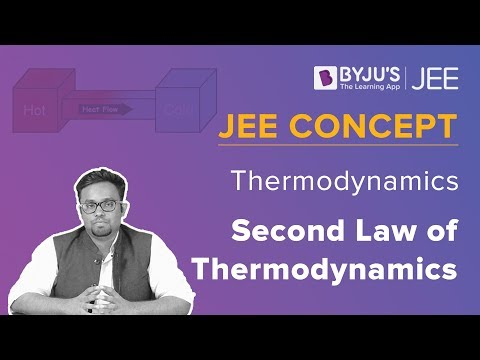## Second Law of Thermodynamics Equation

Mathematically, the second law of thermodynamics is represented as;

ΔSuniv > 0

where ΔSuniv is the change in the entropy of the universe.

Entropy is a measure of the randomness of the system or it is the measure of energy or chaos within an isolated system. It can be considered as a quantitative index that describes the quality of energy.

Meanwhile, there are few factors that cause an increase in entropy of the closed system. Firstly, in a closed system, while the mass remains constant there is an exchange of heat with the surroundings. This change in the heat content creates a disturbance in the system thereby increasing the entropy of the system.

Secondly, internal changes may occur in the movements of the molecules of the system. This leads to disturbances which further causes irreversibilities inside the system resulting in the increment of its entropy.

Also Read: Third Law of Thermodynamics

## Different Statements of The Law

There are two statements on the second law of thermodynamics which are;

1. Kelvin- Plank Statement
2. Clausius Statement

### Kelvin-Planck Statement

It is impossible for a heat engine to produce a network in a complete cycle if it exchanges heat only with bodies at a single fixed temperature.

Exceptions:

If Q2 =0 (i.e., Wnet = Q1, or efficiency=1.00), the heat engine produces work in a complete cycle by exchanging heat with only one reservoir, thus violating the Kelvin-Planck statement.

Also Read: Zeroth Law Of Thermodynamics

### Clausius’s Statement

It is impossible to construct a device operating in a cycle that can transfer heat from a colder body to warmer without consuming any work.

In other words, unless the compressor is driven by an external source, the refrigerator won’t be able to operate.

Heat pump and Refrigerator works on Clausius’s statement.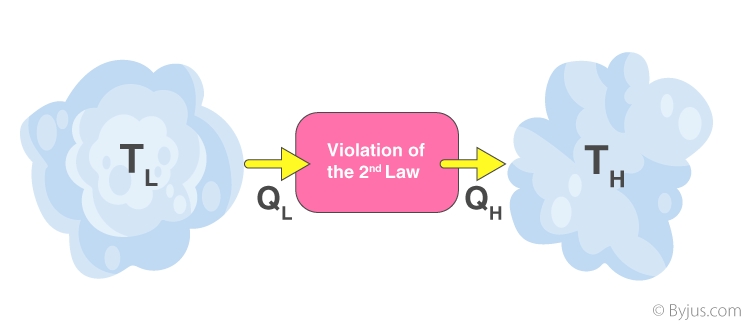Both Clausius’s and Kelvin’s statements are equivalent i.e a device violating Clausius’s statement will also violate Kelvin’s statement and vice versa.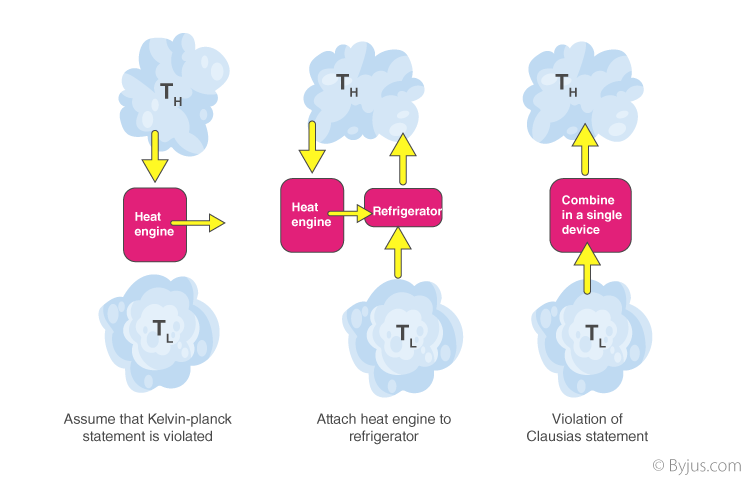In addition to these statements, a French physicist named Nicolas Léonard Sadi Carnot also known as”father of thermodynamics,” basically introduced the Second Law of Thermodynamics. However, as per his statement, he emphasized the use of caloric theory for the description of the law. Caloric (self repellent fluid) relates to heat and Carnot observed that some caloric was lost in the motion cycle.

## Perpetual Motion Machine of the Second Kind (PMM2)

The device that produces work while interacting with a single heat reservoir is known as a perpetual motion machine of the second kind (PMM2). Also, a device that violates the second law of thermodynamics is a perpetual motion machine of the second kind.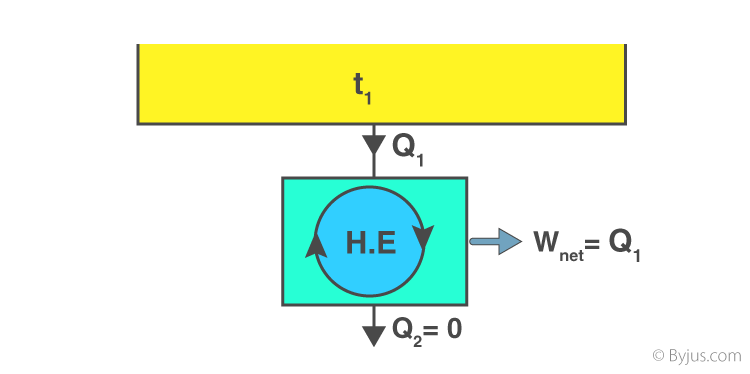Thus, a heat engine has to interact with at least two thermal reservoirs at different temperatures to produce work in a cycle. So long as there is a difference in temperature, motive power (i.e., work) can be produced. If the bodies with which the heat engine exchange heat are of finite heat capacities, work will be produced by the heat engine until the temperature of the two bodies is equalised.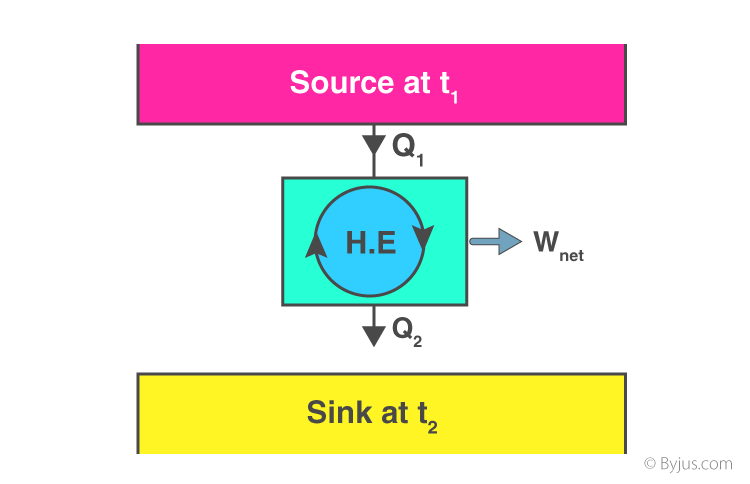## Solved Examples of Second Law Of Thermodynamics

1. A heat pump uses 300 J of work to remove 400 J of heat from the low-temperature reservoir. How much heat is delivered to a higher temperature reservoir?

Solution:

W = 300 J

Q= 400 J

QH = WQC

QH = 300 J + 400 J

QH = 700 J

Heat delivered to the higher temperature reservoir is 700 J.

2. A reversible heat engine receives 4000 KJ of heat from a constant temperature source at 600 K . If the surroundings is at 300K. determine the (a) the availability of heat energy, (b) Unavailable heat.

Solution:

Q1 = 4000 KJ

T1 = 600K

T0 = 300 K

$Change in entropy\left ( \Delta S \right ) = \int \frac{dQ}{T}$ $\Delta S = \frac{Q_{1}}{\left ( T_{1}+T_{0} \right )}$

Change in entropy = 4.44 KJ/K

The availability of heat energy,

A = Q1 – T0(ΔS)

A = 4000 – 300(4.44)

A = 2,668 KJ.

Unavailable heat (U.A) = T0 (ΔS)

(U.A) = 300 (4.44)

(U.A) = 1332 KJ.

(a) the availability of heat energy (A) = 2668 KJ

(b)Unavailable heat (U.A) = 1332 KJ